# (11) Kepler's First Law of Planetary Motion

The laws of orbital motion are mathematical, and one cannot explore them without some mathematics. The math used here is rather elementary; if you need a refresher click here. Otherwise, you can just skip the equations and follow the narrative.

## The Mathematical Description of a Curve

As already noted, the cartesian system labels any point in a plane (e. g. on a flat sheet of paper) by a pair of numbers (x,y), its distance from two perpendicular axes. These numbers are known as the "coordinates" of the point.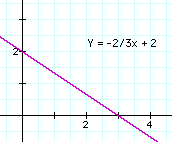A line in the plane--straight or curved--contains many points, each with its own (x,y) coordinates. Often there exists a formula ("equation") which connects x and y: for instance, straight lines have a relationship

y = ax + b

where any pair of numbers (a,b), positive, negative or zero, gives some straight line. More complicated relationships give curves: for instance

y = ax2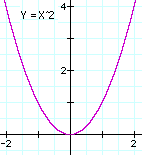gives a parabola, with a any number. Usually (though not always) y is isolated, so that the formula has the form

y = y(x)

where y(x) stands for "any expression involving x" or in mathematical terms, a "function of x." The curves drawn here are the straight line y = -(2/3)x + 2 and the parabola y = x2. A list of some of their points follows.

Straight line:

 x -1 0 1 2 3 4 y 8/3 2 4/3 2/3 0 -2/3

Parabola:

 x -2 -1.5 -1 -0.5 0 0.5 1 1.5 2 y 4 2.25 1 0.25 0 0.25 1 2.25 4

## Polar Coordinates

As already noted, other ways exist for labeling points in the plane. For instance, a point may be labeled by its distance r from a central point O ("origin") and the angle f (or Greek f) which the line OP makes with some standard direction. Such "polar coordinates" (drawing on the left, below) are the ones best suited for describing planetary motion.

Again, if all the values of (r,f) of a curve are related by some equation which can be symbolically written

r = r(f)

then the function r(f) is said to be the equation of the line, in polar coordinates. The simplest function is a constant number a, giving the line

r = a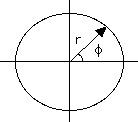The value of r equals a for any value of f. That gives a circle around the origin, its radius equal to a, shown in the drawing on the right above.

## The Ellipse

Consider next the curve whose equation is

r = a(1- e2)/ (1+ e cos f)

where the eccentricity e is a number between 0 and 1. If e = 0, this is clearly the circle encountered earlier.

How about other values? The function cos f represents a wave-like behavior (picture below), and as f goes through a full circle, it goes down, from +1 to 0, then -1, then up again to 0 and +1. The denominator also rises and falls as a wave, and it is smallest when cos f = -1 . Here is the table of the main values (360 is in parentheses, because it represents the same direction as 0 degrees):f degrees 0 90 180 270 (360) cos f 1 0 -1 0 1 1 + cos f 1 + e 1 1 - e 1 1 + e
 As long as e is less than 1, the denominator is always positive. It is never zero, so that for any f one can name, one can always find a suitable r. In other words, the curve goes completely around the origin, it is closed. The expression (1 - e2) can be factored--that is, written as two expressions multiplied by each other ("the product of two expressions"). As discussed in the section on algebraic identities 1 - e2= (1 - e)(1 + e) At some of the points on the above table, one of the expressions cancels the denominator, giving:
 f degrees 0 90 180 270 (360) r a(1 - e) a(1 - e2) a(1 + e) a(1 - e2) a(1 - e)

Next Stop: #12 Kepler's Second Law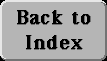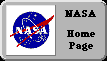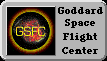Author and curator: David P. Stern, u5dps@lepvax.gsfc.nasa.gov
Last updated 6 March 1999